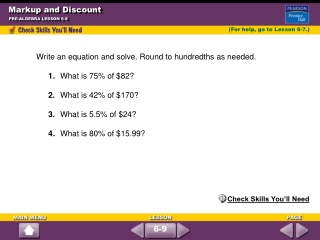DownloadDownload PresentationMarkup and Discount

# Markup and Discount

Télécharger la présentation## Markup and Discount

- - - - - - - - - - - - - - - - - - - - - - - - - - - E N D - - - - - - - - - - - - - - - - - - - - - - - - - - -
##### Presentation Transcript

1. Markup and Discount PRE-ALGEBRA LESSON 6-9 (For help, go to Lesson 6-7.) Write an equation and solve. Round to hundredths as needed. 1. What is 75% of \$82? 2. What is 42% of \$170? 3. What is 5.5% of \$24? 4. What is 80% of \$15.99? Check Skills You’ll Need 6-9

2. Markup and Discount PRE-ALGEBRA LESSON 6-9 Solutions 1. What is 75% of \$82? 2. What is 42% of \$170? n = 0.75 • 82 n = 0.42 • 170 n = 61.5 n = 71.4 75% of \$82 is \$61.50. 42% of \$170 is \$71.40. 3. What is 5.5% of \$24? 4. What is 80% of \$15.99? n = 0.055 • 24 n = 0.80 • 15.99 n = 1.32 n = 12.79 5.5% of \$24 is \$1.32. 80% of \$15.99 is \$12.79. 6-9

3. Markup and Discount PRE-ALGEBRA LESSON 6-9 Vocabulary _________ is the amount of increase in price. Percent of Increase is _______________________ _________________________________________ _____________ is the amount of price decrease. Percent of discount is ________________________ _________________________________________ 6-9

4. Markup and Discount PRE-ALGEBRA LESSON 6-9 A grocery store has a 20% markup on a can of soup. The can of soup costs the store \$1.25. Find the markup. markup = percent of markup • store’s cost = 0.2 • 1.25 = 0.25        Simplify. The markup is \$.25. Quick Check 6-9

5. Markup and Discount PRE-ALGEBRA LESSON 6-9 A bookstore pays \$4.60 for a novel. The percent of markup is 45%. Find the novel’s selling price. 0.45 • 4.60 = 2.07 Multiply to find the markup. 4.60 + 2.07 = 6.53   Store’s cost + markup = selling price. The selling price is \$6.67. Quick Check 6-9

6. Markup and Discount PRE-ALGEBRA LESSON 6-9 A camera that regularly sells for \$210 is on sale for 30% off. Find the discount. discount = percent of discount • regular price = 0.30 • 210 = 63 The discount is \$63. Quick Check 6-9

7. Markup and Discount PRE-ALGEBRA LESSON 6-9 Solve. 1. The school store has a 65% markup on each stapler. Each stapler costs the store \$2.10. Find the markup. 2. A clothes store pays \$40 for a skirt. The percent of markup is 25%. Find the skirt’s selling price. 3. A pair of shoes that regularly sells for \$94.99 is on sale for 30% off. What is the sale price? \$1.37 \$50 \$66.49 6-9